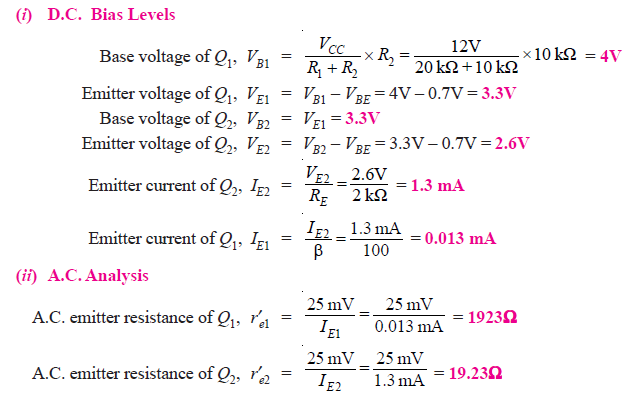# Solved Problems on Amplifiers with Negative Feedback

Q1. The voltage gain of an amplifier without feedback is 3000. Calculate the voltage gain of the amplifier if negative voltage feedback is introduced in the circuit. Given that feedback fraction mv = 0.01.

Solution :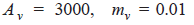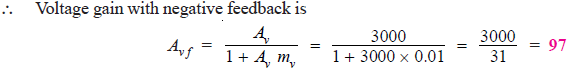Q2. The overall gain of a multistage amplifier is 140. When negative voltage feedback is applied, the gain is reduced to 17.5. Find the fraction of the output that is fedback to the input.

Solution :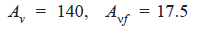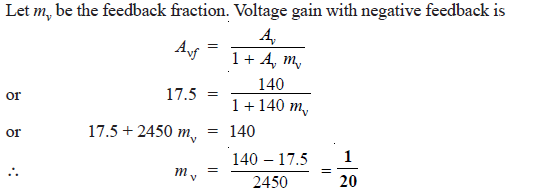Q3. When negative voltage feedback is applied to an amplifier of gain 100, the overall gain falls to 50.
(i) Calculate the fraction of the output voltage fedback.
(ii) If this fraction is maintained, calculate the value of the amplifier gain required if the overall stage gain is to be 75.

Solution :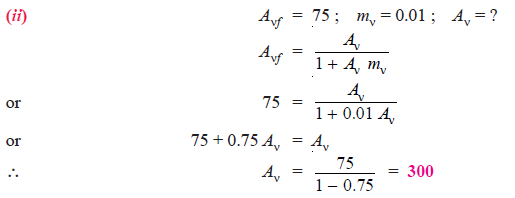Q4. With a negative voltage feedback, an amplifier gives an output of 10 V with an input of 0.5 V. When feedback is removed, it requires 0.25 V input for the same output. Calculate (i) gain without feedback (ii) feedback fraction m.

Solution :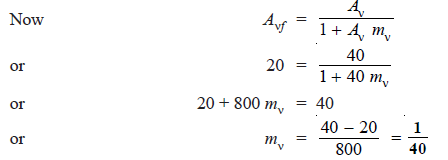Q5. The gain of an amplifier without feedback is 50 whereas with negative voltage feedback, it falls to 25. If due to ageing, the amplifier gain falls to 40, find the percentage reduction in stage gain (i) without feedback and (ii) with negative feedback.

Solution :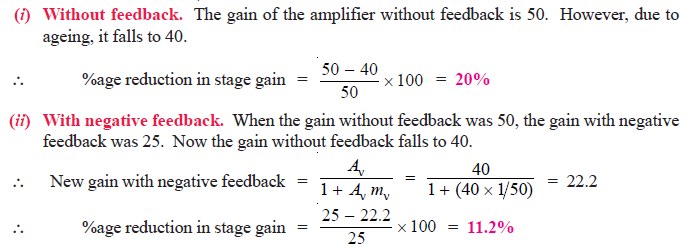Q6. An amplifier has a voltage amplification Av and a fraction mof its output is fedback in opposition to the input. If m= 0.1 and Aν = 100, calculate the percentage change in the gain of the system if Aν falls 6 db due to ageing.

Solution :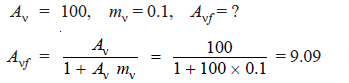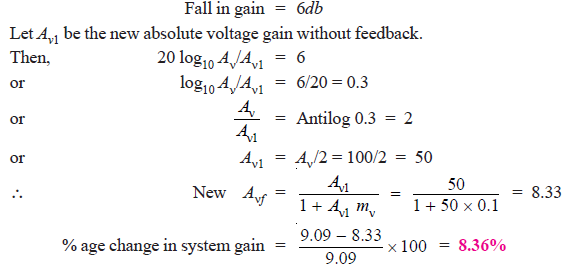Q7. An amplifier has a voltage gain of 500 without feedback. If a negative feedback is applied, the gain is reduced to 100. Calculate the fraction of the output fed back. If, due to ageing of components, the gain without feedback falls by 20%, calculate the percentage fall in gain with feedback.

Solution :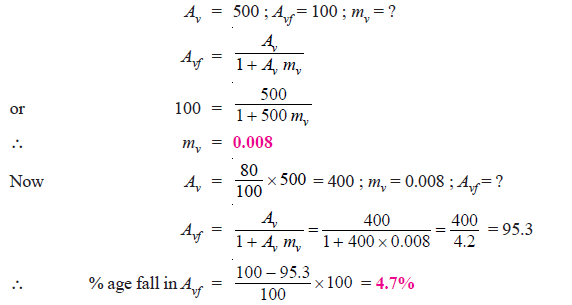Note that without negative feedback, the change in gain is 20%. However, when negative feedback is applied, the change in gain (4.7%) is much less. This shows that negative feedback provides voltage gain stability.

Q8. An amplifier has an open-loop gain Av = 100,000. A negative feedback of 10 db is applied. Find (i) voltage gain with feedback (ii) value of feedback fraction m.

Solution :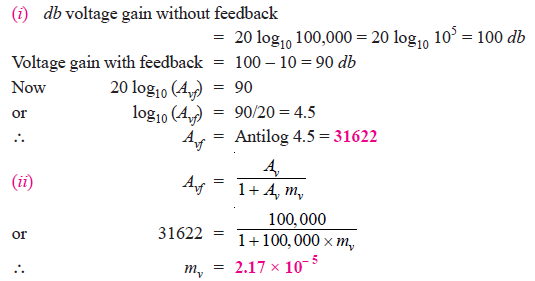Q9. An amplifier with an open-circuit voltage gain of 1000 has an output resistance of 100 Ω and feeds a resistive load of 900 Ω. Negative voltage feedback is provided by connecting a resistive voltage divider across the output and one-fiftieth of the output voltage is fedback in series with the input signal. Determine the voltage gain with negative feedback.

Solution :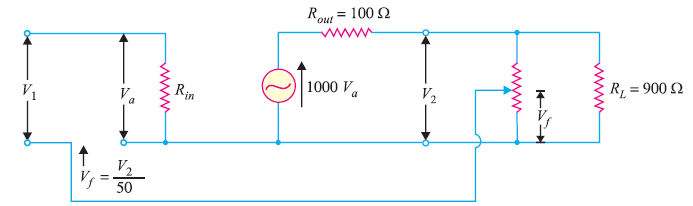Fig. 1

Fig. 1 shows the equivalent circuit of an amplifier along with the feedback circuit.

Voltage gain of the amplifier without feedback is: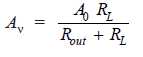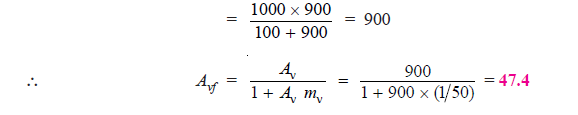Q10. An amplifier is required with a voltage gain of 100 which does not vary by more than 1%. If it is to use negative feedback with a basic amplifier the voltage gain of which can vary by 20%, determine the minimum voltage gain required and the feedback factor.

Solution :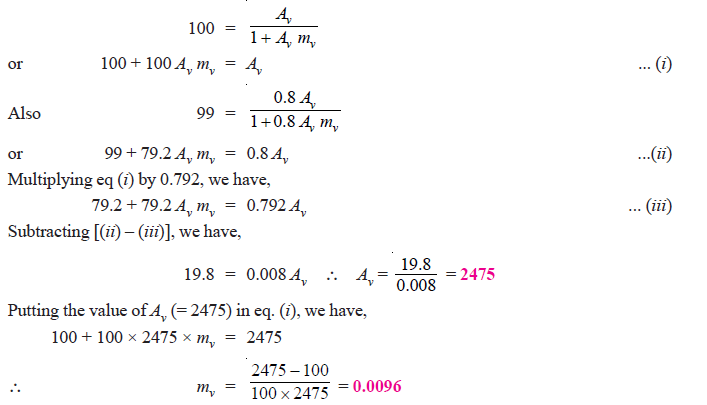Q11. Fig. 2 shows the negative voltage feedback amplifier. If the gain of the amplifier without feedback is 10,000, find : (i) feedback fraction (ii) overall voltage gain (iii) output voltage if input voltage is 1 mV.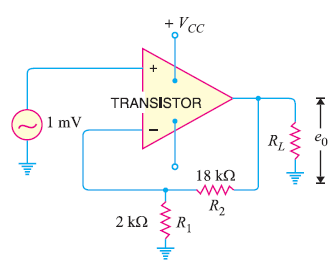Fig. 2

Solution :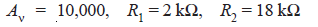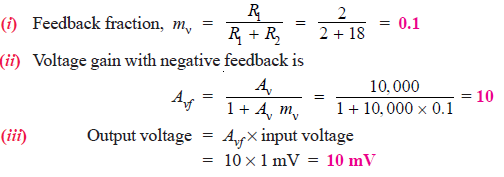Q12. Fig. 3 shows the circuit of a negative voltage feedback amplifier. If without feedback, Av = 10,000, Zin = 10 kΩ, Zout = 100 Ω, find :(i) feedback fraction (ii) gain with feedback (iii) input impedance with feedback (iv) output impedance with  feedback.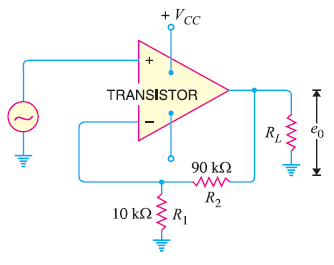Fig. 3

Solution :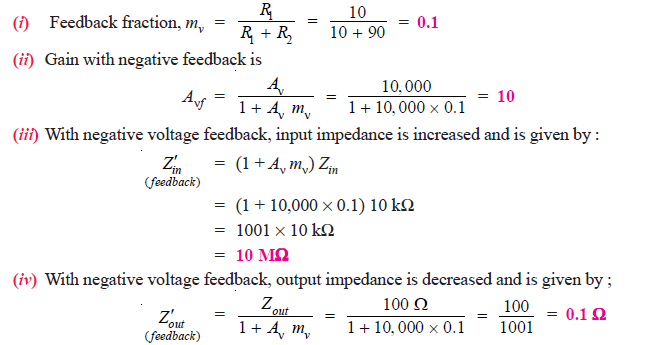Q13. The gain and distortion of an amplifier are 150 and 5% respectively without feedback. If the stage has 10% of its output voltage applied as negative feedback, find the distortion of the amplifier with feedback.

Solution :

Gain without feedback, Aν = 150
Distortion without feedback, D = 5% = 0.05
Feedback fraction, mv = 10% = 0.1
If Dvf  is the distortion with negative feedback, then,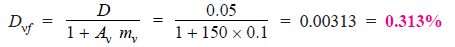It may be seen that by the application of negative voltage feedback, the amplifier distortion is reduced from 5% to 0.313%.

Q14. An amplifier has a gain of 1000 without feedback and cut-off frequencies are f1 = 1.5 kHz and f2 = 501.5 kHz. If 1% of output voltage of the amplifier is applied as negative feedback, what are the new cut-off frequencies ?

Solution :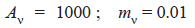The new lower cut-off frequency with feedback is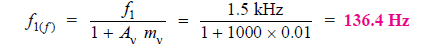The new upper cut-off frequency with feedback is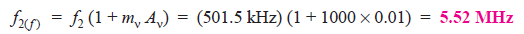Note the effect of negative voltage feedback on the bandwidth of the amplifier. The lower cut-off frequency is decreased by a factor (1 + mν Aν) while upper cut-off frequency is increased by a factor (1 + mν Aν). In other words, the bandwidth of the amplifier is increased approximately by a factor (1 + mν Aν).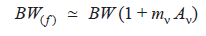where BW = Bandwidth of the amplifier without feedback
BW(f) = Bandwidth of the amplifier with negative feedback

Q15. The current gain of an amplifier is 200 without feedback. When negative current feedback is applied, determine the effective current gain of the amplifier. Given that current attenuation mi = 0.012.

Solution :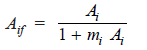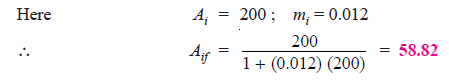Q16. An amplifier has a current gain of 240 and input impedance of 15 kΩ without feedback. If negative current feedback (mi = 0.015) is applied, what will be the input impedance of the amplifier ?

Solution :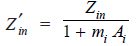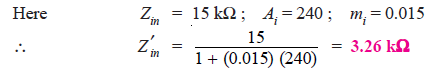Q17. An amplifier has a current gain of 200 and output impedance of 3 kΩ without feedback. If negative current feedback (mi = 0.01) is applied; what is the output impedance of the amplifier ?

Solution :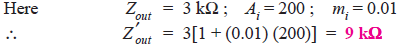Q18. An amplifier has a current gain of 250 and a bandwidth of 400 kHz without feedback. If negative current feedback (m= 0.01) is applied, what is the bandwidth of the amplifier?

Solution :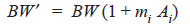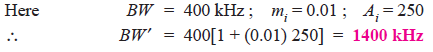Q19. For the emitter follower circuit shown in Fig. 4, find Vand IE. Also draw the dc load line for this circuit.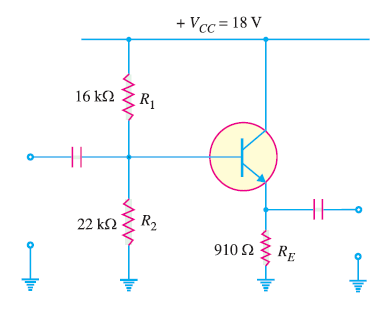Fig. 4

Solution :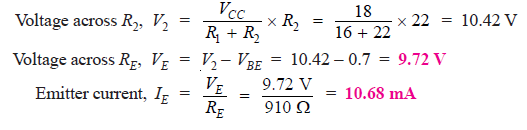By joining points A and B, d.c. load line AB is constructed [See Fig. 5].Fig. 5

Q20. Determine the voltage gain of the emitter follower circuit shown in Fig. 6.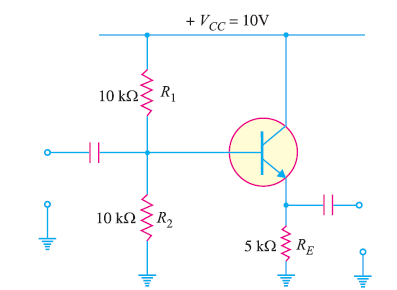Fig. 6

Solution :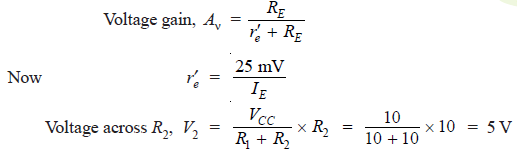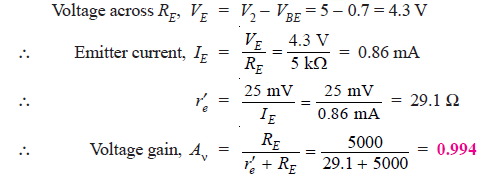Q21. If in the above example, a load of 5 kΩ is added to the emitter follower, what will be the voltage gain of the circuit ?

Solution :

When a load of 5 kΩ is added to the emitter follower, the circuit becomes as shown in Fig. 7.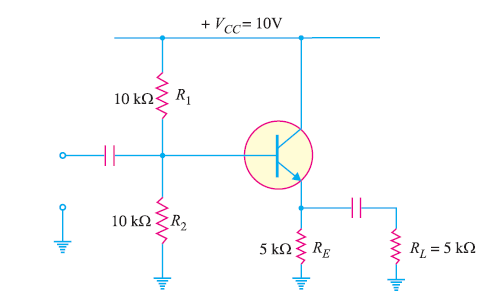Fig. 7

The coupling capacitor acts as a short for a.c. signal so that RE and Rare in parallel.
Therefore, the external emitter resistance RE changes to R’E whereQ22. For the emitter follower circuit shown in Fig. 8, find the input impedance.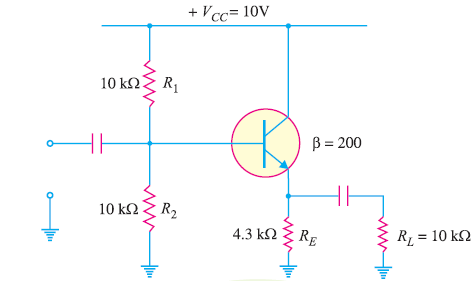Fig. 8

Solution :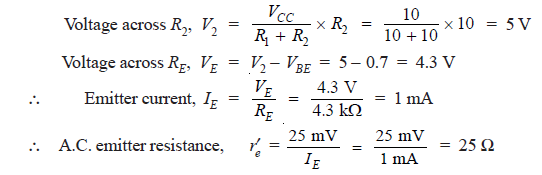Q23. Determine the output impedance of the emitter follower shown in Fig. 9. Given that r’e = 20 Ω.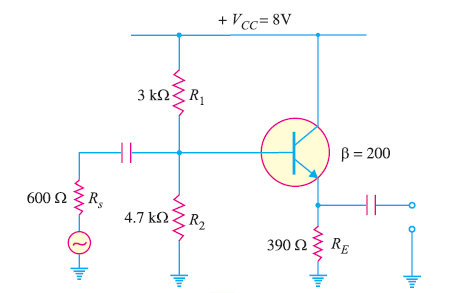Fig.9

Solution :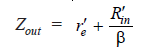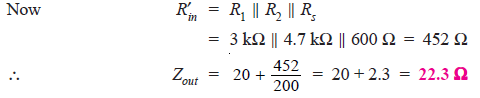Note that output impedance of the emitter follower is very low. On the other hand, it has high input impedance. This property makes the emitter follower a perfect circuit for connecting a low impedance load to a high-impedance source.

Q24. Determine (i) d.c. value of current in RE (ii) input impedance of the Darlington amplifier shown in Fig. 10.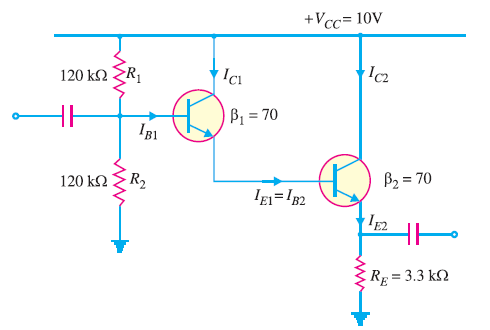Fig. 10

Solution :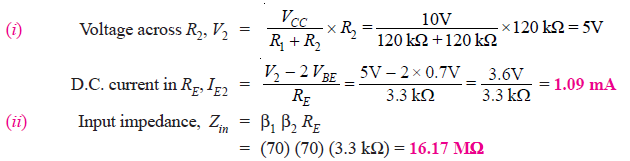Q25. For the Darlington amplifier in Fig. 11, find (i) the d.c. levels of both the transistors and (ii) a.c. emitter resistances of both transistors.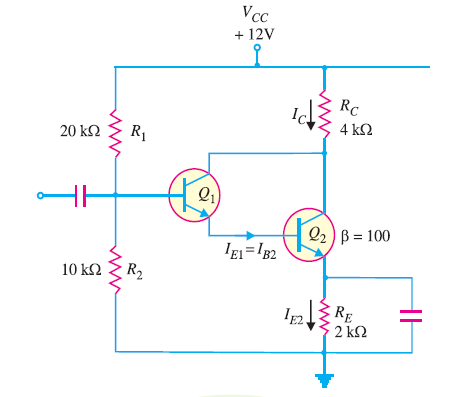Fig. 11

Solution :